Trigonometric Functions Graphing the Trigonometric Function Graphing Trigonometric

• Slides: 52Trigonometric Functions Graphing the Trigonometric Function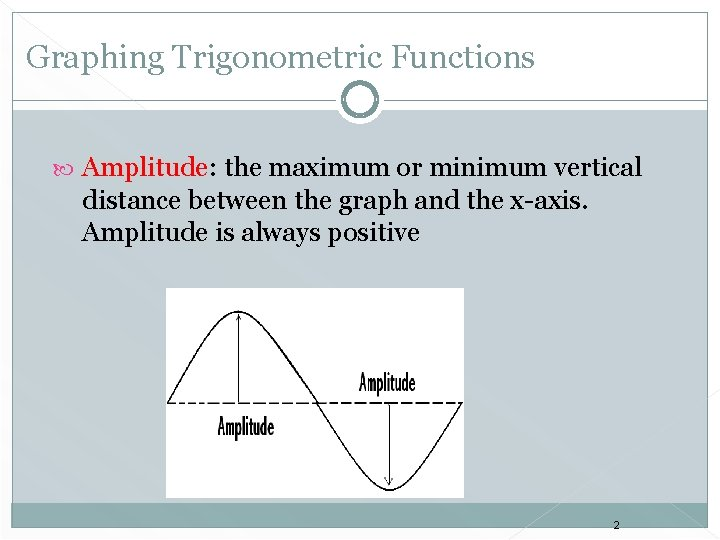Graphing Trigonometric Functions Amplitude: the maximum or minimum vertical distance between the graph and the x-axis. Amplitude is always positive 2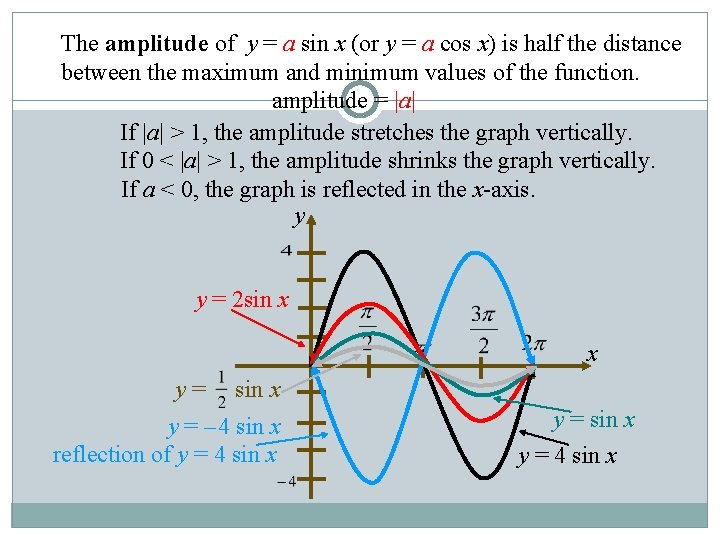The amplitude of y = a sin x (or y = a cos x) is half the distance between the maximum and minimum values of the function. amplitude = |a| If |a| > 1, the amplitude stretches the graph vertically. If 0 < |a| > 1, the amplitude shrinks the graph vertically. If a < 0, the graph is reflected in the x-axis. y y = 2 sin x x y= sin x y = – 4 sin x reflection of y = 4 sin x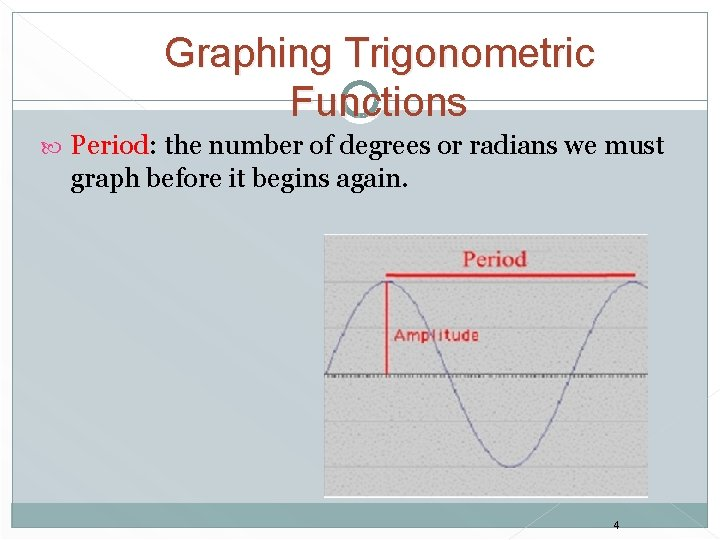Graphing Trigonometric Functions Period: the number of degrees or radians we must graph before it begins again. 4The period of a function is the x interval needed for the function to complete one cycle. For b 0, the period of y = a sin bx is . For b 0, the period of y = a cos bx is also . If 0 < b < 1, the graph of the function is stretched horizontally. y period: 2 period: x If b > 1, the graph of the function is shrunk horizontally. y period: 2 x period: 4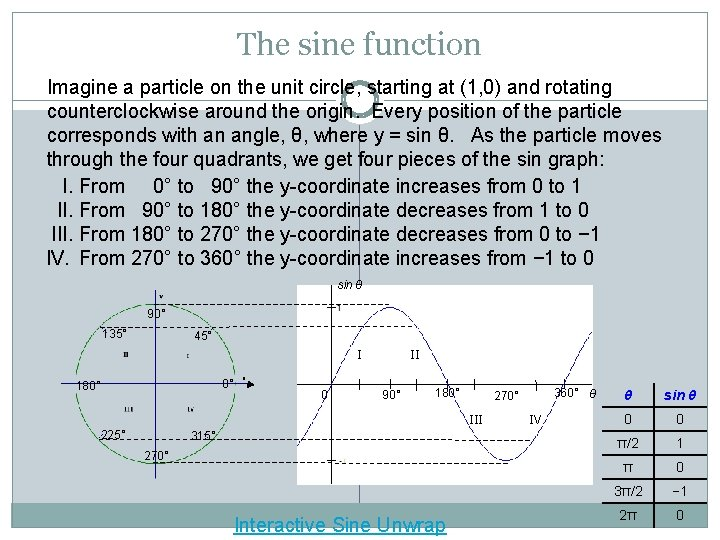The sine function Imagine a particle on the unit circle, starting at (1, 0) and rotating counterclockwise around the origin. Every position of the particle corresponds with an angle, θ, where y = sin θ. As the particle moves through the four quadrants, we get four pieces of the sin graph: I. From 0° to 90° the y-coordinate increases from 0 to 1 II. From 90° to 180° the y-coordinate decreases from 1 to 0 III. From 180° to 270° the y-coordinate decreases from 0 to − 1 IV. From 270° to 360° the y-coordinate increases from − 1 to 0 sin θ 90° 135° 45° I 0° 180° 0 II 90° 180° III 225° 315° 270° Interactive Sine Unwrap 360° θ 270° IV θ sin θ 0 0 π/2 1 π 0 3π/2 − 1 2π 0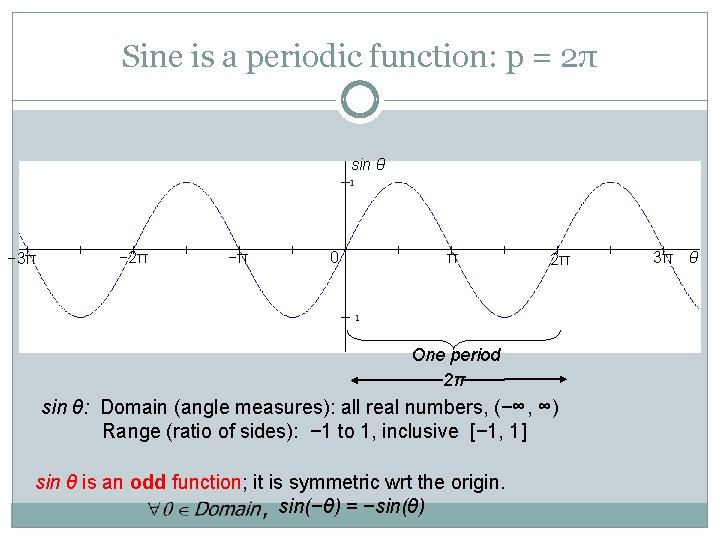Sine is a periodic function: p = 2π sin θ − 3π − 2π −π 0 π 2π One period 2π sin θ: Domain (angle measures): all real numbers, (−∞, ∞) Range (ratio of sides): − 1 to 1, inclusive [− 1, 1] sin θ is an odd function; it is symmetric wrt the origin. sin(−θ) = −sin(θ) 3π θGraph of the Sine Function To sketch the graph of y = sin x first locate the key points. These are the maximum points, the minimum points, and the intercepts. x 0 sin x 0 1 0 -1 0 Then, connect the points on the graph with a smooth curve that extends in both directions beyond the five points. A single cycle is called a period. y = sin x y x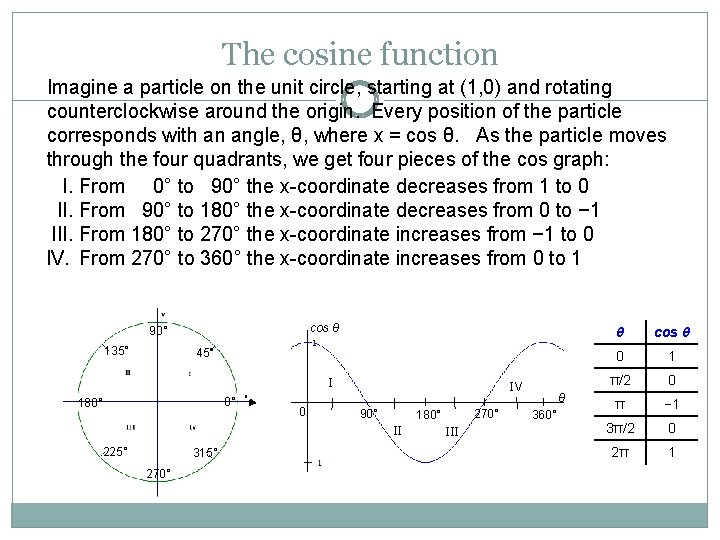The cosine function Imagine a particle on the unit circle, starting at (1, 0) and rotating counterclockwise around the origin. Every position of the particle corresponds with an angle, θ, where x = cos θ. As the particle moves through the four quadrants, we get four pieces of the cos graph: I. From 0° to 90° the x-coordinate decreases from 1 to 0 II. From 90° to 180° the x-coordinate decreases from 0 to − 1 III. From 180° to 270° the x-coordinate increases from − 1 to 0 IV. From 270° to 360° the x-coordinate increases from 0 to 1 cos θ 90° 135° 45° I 0° 180° 0 IV 90° II 225° 315° 270° 180° III θ 360° θ cos θ 0 1 π/2 0 π − 1 3π/2 0 2π 1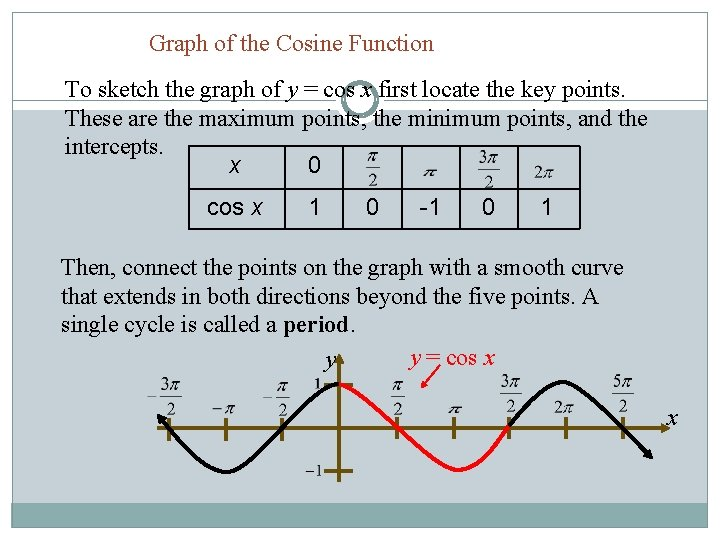Graph of the Cosine Function To sketch the graph of y = cos x first locate the key points. These are the maximum points, the minimum points, and the intercepts. x 0 cos x 1 0 -1 0 1 Then, connect the points on the graph with a smooth curve that extends in both directions beyond the five points. A single cycle is called a period. y = cos x y xCosine is a periodic function: p = 2π cos θ θ − 3π − 2π −π 0 π One period 2π cos θ: Domain (angle measures): all real numbers, (−∞, ∞) Range (ratio of sides): − 1 to 1, inclusive [− 1, 1] cos θ is an even function; it is symmetric wrt the y-axis. cos(−θ) = cos(θ) 2π 3πProperties of Sine and Cosine graphs 1. The domain is the set of real numbers 2. The rage is set of “y” values such that -1≤ y ≤ 1 3. The maximum value is 1 and the minimum value is -1 4. The graph is a smooth curve 5. Each function cycles through all the values of the range over an x interval or 2π 6. The cycle repeats itself identically in both direction of the x-axis 12Sine Graph Given : A sin Bx Amplitude = IAI period = 2π/B Example: y=5 sin 2 X › Amp=5 π/2 π/4 › Period=2π/2 =π 3π/4 π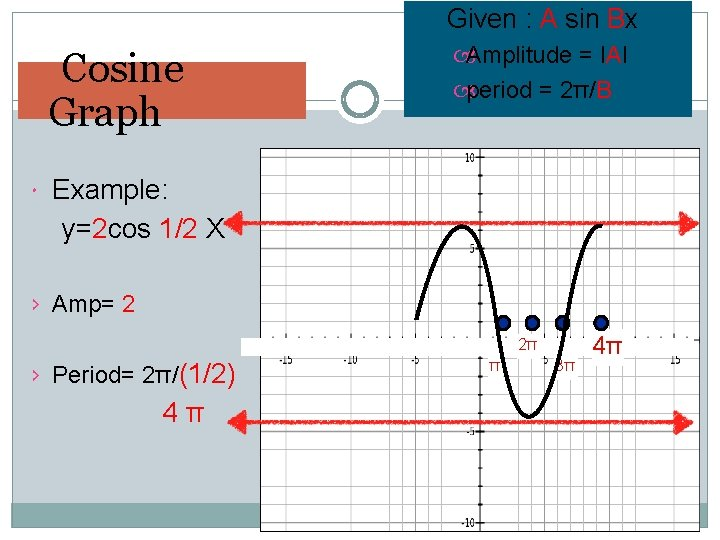Given : A sin Bx Cosine Graph Amplitude = IAI period = 2π/B Example: y=2 cos 1/2 X › Amp= 2 2π › Period= 2π/(1/2) 4π π 3π 4π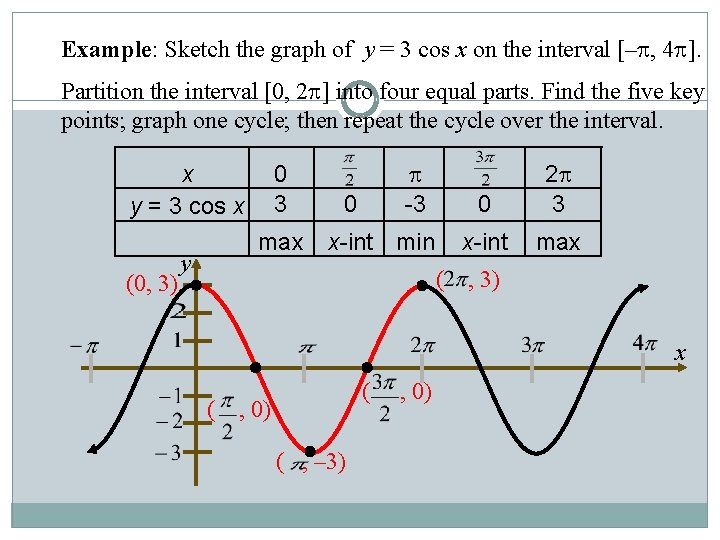Example: Sketch the graph of y = 3 cos x on the interval [– , 4 ]. Partition the interval [0, 2 ] into four equal parts. Find the five key points; graph one cycle; then repeat the cycle over the interval. x y = 3 cos x (0, 3) 0 3 max y -3 0 x-int min ( 0 2 3 x-int max , 3) x ( ( , 0) ( , – 3) , 0)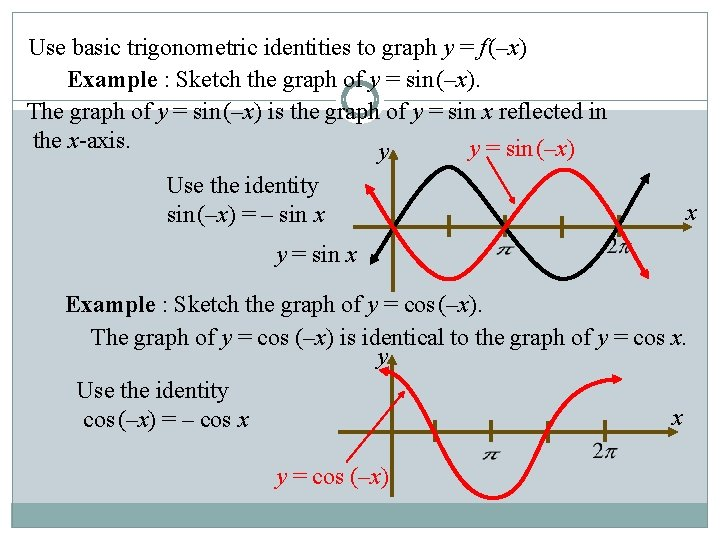Use basic trigonometric identities to graph y = f (–x) Example : Sketch the graph of y = sin (–x). The graph of y = sin (–x) is the graph of y = sin x reflected in the x-axis. y = sin (–x) y Use the identity sin (–x) = – sin x x y = sin x Example : Sketch the graph of y = cos (–x). The graph of y = cos (–x) is identical to the graph of y = cos x. y Use the identity x cos (–x) = – cos x y = cos (–x)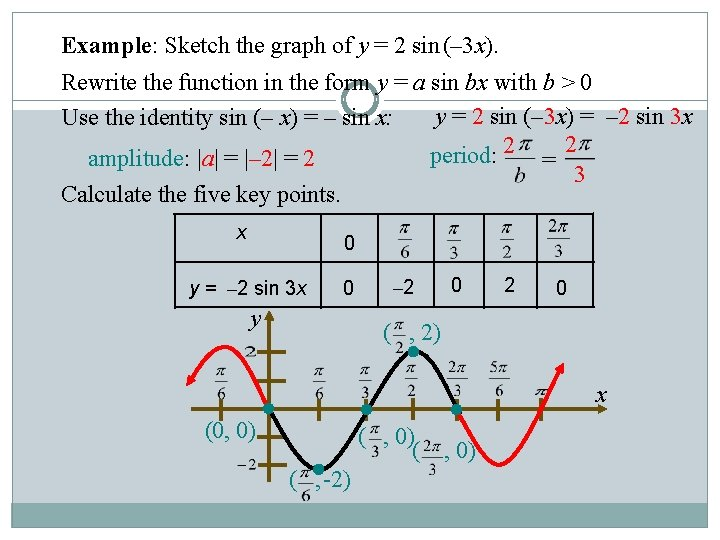Example: Sketch the graph of y = 2 sin (– 3 x). Rewrite the function in the form y = a sin bx with b > 0 y = 2 sin (– 3 x) = – 2 sin 3 x Use the identity sin (– x) = – sin x: period: 2 = 2 amplitude: |a| = |– 2| = 2 3 Calculate the five key points. x 0 y = – 2 sin 3 x 0 y – 2 0 ( , 2) x (0, 0) ( ( , -2) , 0)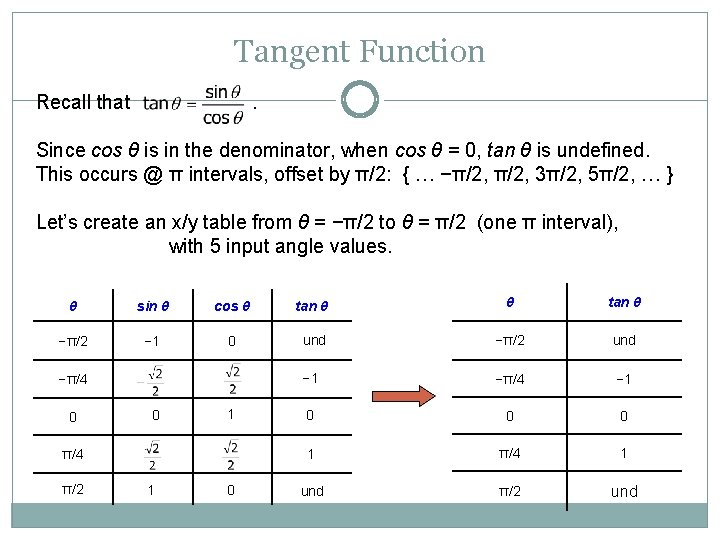Tangent Function Recall that . Since cos θ is in the denominator, when cos θ = 0, tan θ is undefined. This occurs @ π intervals, offset by π/2: { … −π/2, 3π/2, 5π/2, … } Let’s create an x/y table from θ = −π/2 to θ = π/2 (one π interval), with 5 input angle values. θ sin θ cos θ tan θ −π/2 − 1 0 und −π/2 und − 1 −π/4 − 1 0 0 0 1 π/4 1 und π/2 und −π/4 0 0 1 π/4 π/2 1 0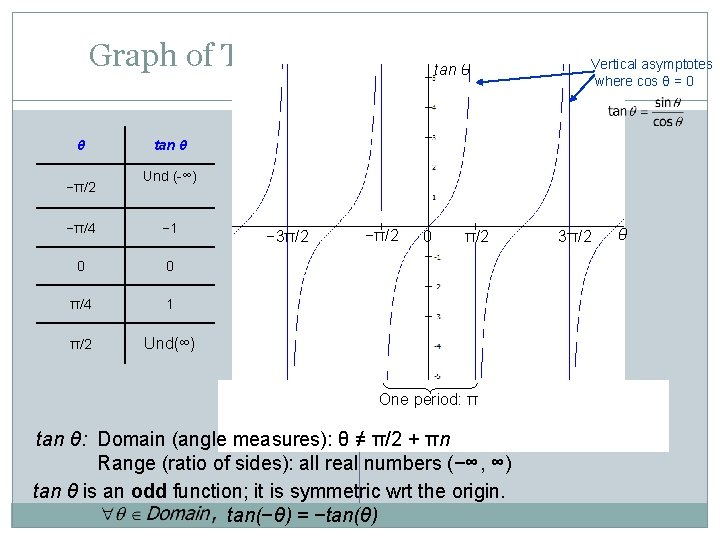Graph of Tangent Function: Periodic Vertical asymptotes tan θ where cos θ = 0 θ −π/2 tan θ Und (-∞) −π/4 − 1 0 0 π/4 1 π/2 Und(∞) − 3π/2 −π/2 0 π/2 One period: π tan θ: Domain (angle measures): θ ≠ π/2 + πn Range (ratio of sides): all real numbers (−∞, ∞) tan θ is an odd function; it is symmetric wrt the origin. tan(−θ) = −tan(θ) 3π/2 θ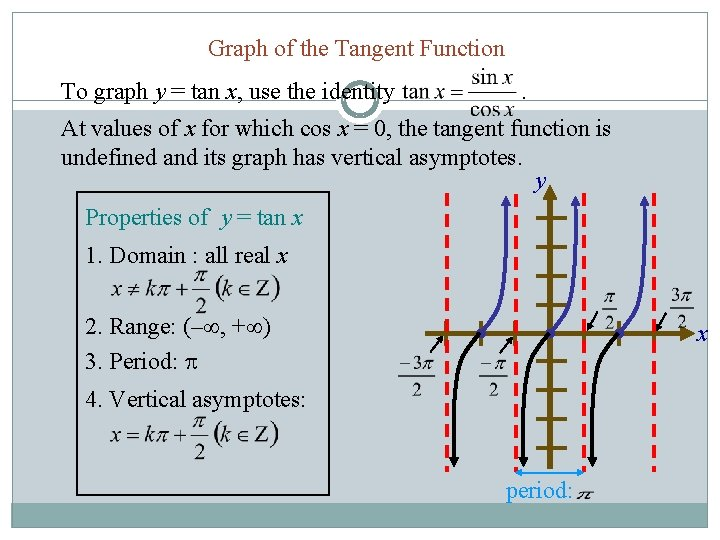Graph of the Tangent Function To graph y = tan x, use the identity . At values of x for which cos x = 0, the tangent function is undefined and its graph has vertical asymptotes. y Properties of y = tan x 1. Domain : all real x 2. Range: (– , + ) 3. Period: x 4. Vertical asymptotes: period: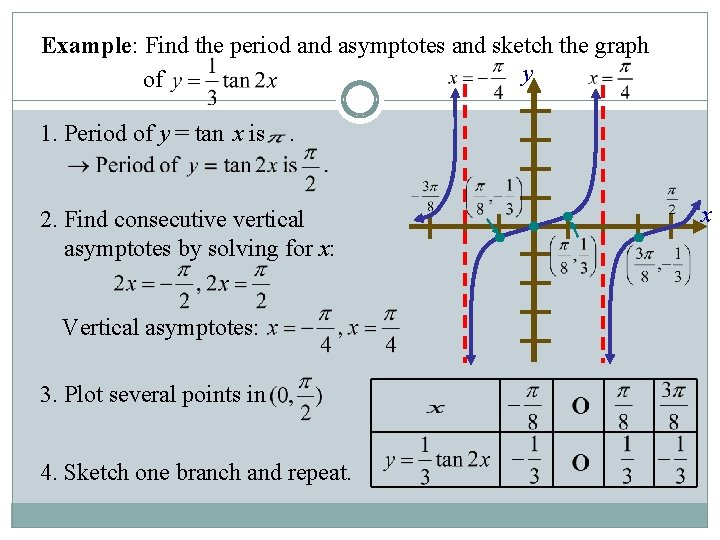Example: Find the period and asymptotes and sketch the graph y of 1. Period of y = tan x is . 2. Find consecutive vertical asymptotes by solving for x: Vertical asymptotes: 3. Plot several points in 4. Sketch one branch and repeat. x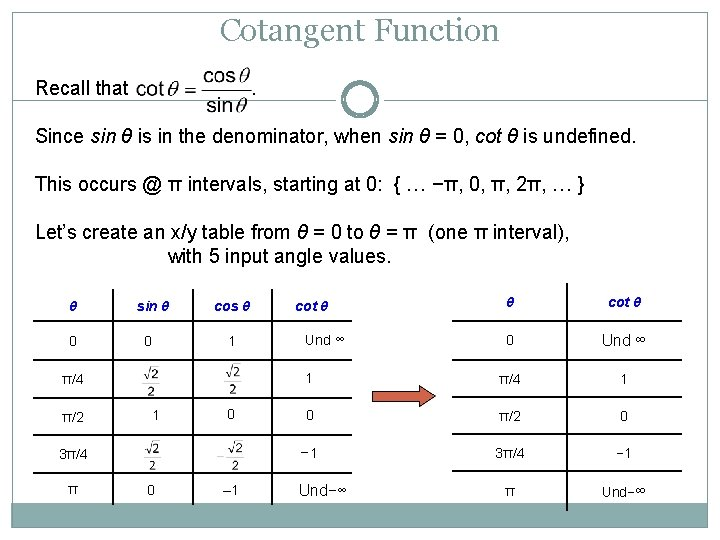Cotangent Function Recall that . Since sin θ is in the denominator, when sin θ = 0, cot θ is undefined. This occurs @ π intervals, starting at 0: { … −π, 0, π, 2π, … } Let’s create an x/y table from θ = 0 to θ = π (one π interval), with 5 input angle values. θ 0 sin θ cos θ 1 0 π/4 π/2 1 0 3π/4 π 0 – 1 θ cot θ 0 Und ∞ 1 π/4 1 0 π/2 0 − 1 3π/4 − 1 π Und−∞ cot θ Und ∞ Und−∞Graph of Cotangent Function: Periodic Vertical asymptotes where sin θ = 0 cot θ θ cot θ 0 ∞ π/4 1 π/2 0 3π/4 − 1 π −∞ − 3π/2 -π −π/2 cot θ: Domain (angle measures): θ ≠ πn Range (ratio of sides): all real numbers (−∞, ∞) cot θ is an odd function; it is symmetric wrt the origin. tan(−θ) = −tan(θ) π 3π/2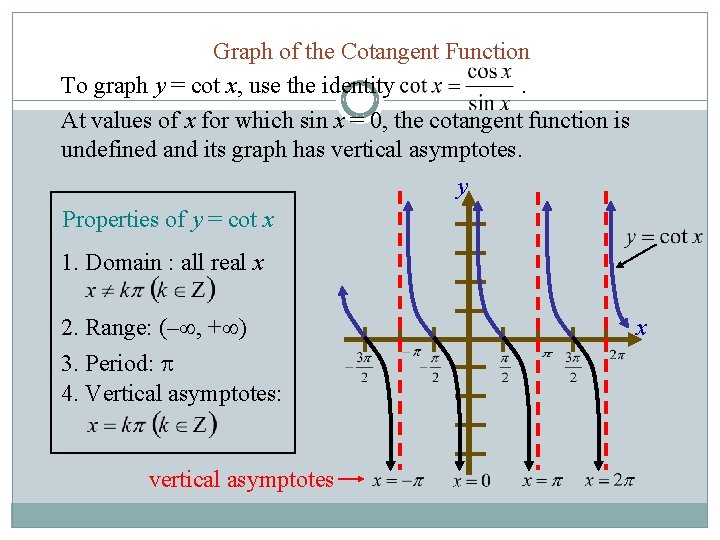Graph of the Cotangent Function To graph y = cot x, use the identity. At values of x for which sin x = 0, the cotangent function is undefined and its graph has vertical asymptotes. y Properties of y = cot x 1. Domain : all real x 2. Range: (– , + ) 3. Period: 4. Vertical asymptotes: vertical asymptotes x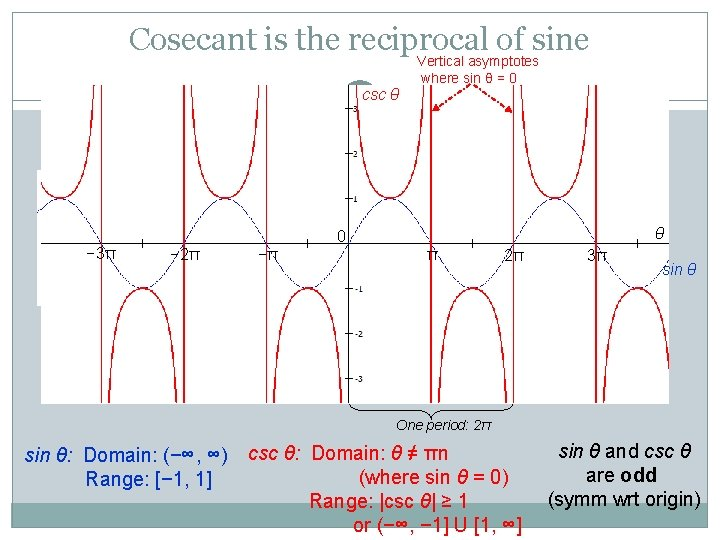Cosecant is the reciprocal of sine csc θ − 3π Vertical asymptotes where sin θ = 0 θ 0 − 2π −π π 2π 3π sin θ One period: 2π sin θ: Domain: (−∞, ∞) Range: [− 1, 1] csc θ: Domain: θ ≠ πn (where sin θ = 0) Range: |csc θ| ≥ 1 or (−∞, − 1] U [1, ∞] sin θ and csc θ are odd (symm wrt origin)Graph of the Cosecant Function To graph y = csc x, use the identity . At values of x for which sin x = 0, the cosecant function is undefined and its graph has vertical asymptotes. y Properties of y = csc x 1. domain : all real x 2. range: (– , – 1] [1, + ) 3. period: 4. vertical asymptotes: where sine is zero. x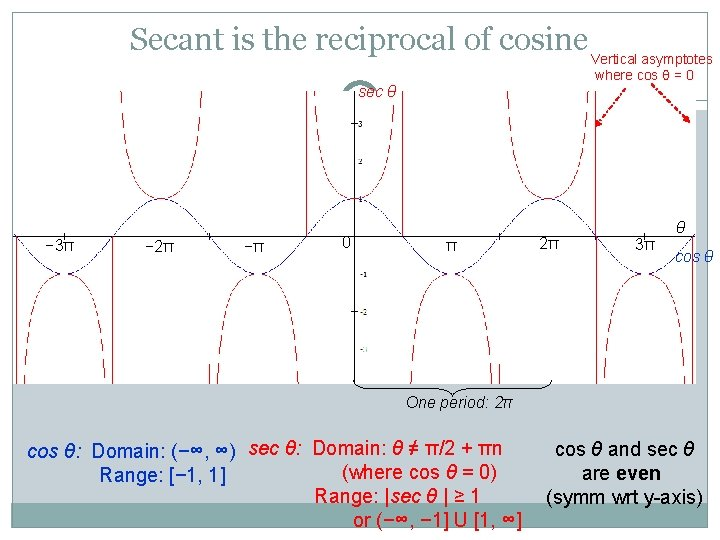Secant is the reciprocal of cosine Vertical asymptotes where cos θ = 0 sec θ − 3π − 2π −π 0 π 2π θ 3π cos θ One period: 2π cos θ: Domain: (−∞, ∞) sec θ: Domain: θ ≠ π/2 + πn (where cos θ = 0) Range: [− 1, 1] Range: |sec θ | ≥ 1 or (−∞, − 1] U [1, ∞] cos θ and sec θ are even (symm wrt y-axis)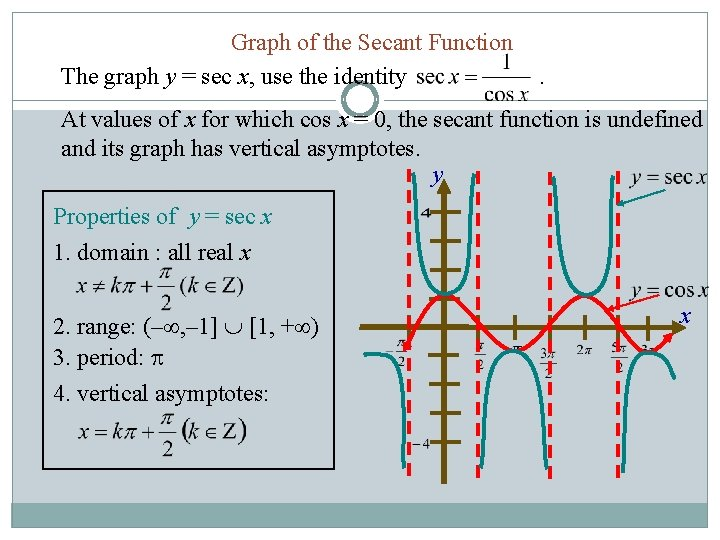Graph of the Secant Function The graph y = sec x, use the identity . At values of x for which cos x = 0, the secant function is undefined and its graph has vertical asymptotes. y Properties of y = sec x 1. domain : all real x 2. range: (– , – 1] [1, + ) 3. period: 4. vertical asymptotes: xSummary of Graph Characteristics Def’n ∆ sin θ csc θ cos θ sec θ tan θ cot θ о Period Domain Range Even/Odd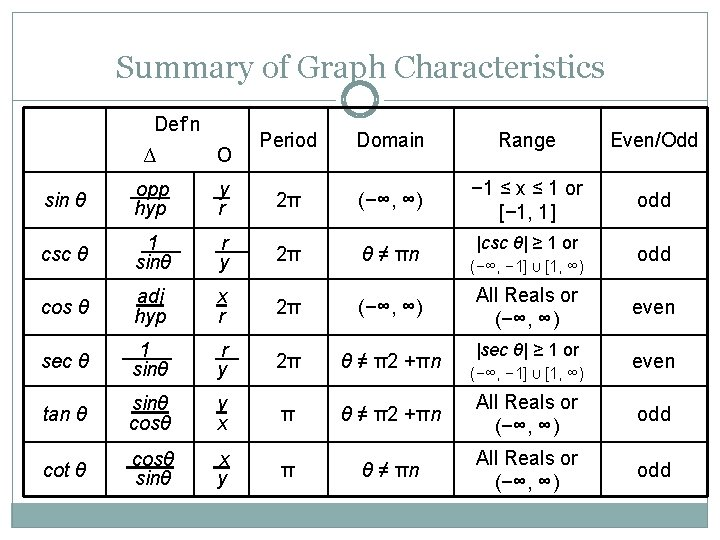Summary of Graph Characteristics Def’n Period Domain Range Even/Odd − 1 ≤ x ≤ 1 or [− 1, 1] odd ∆ о sin θ opp hyp y r 2π (−∞, ∞) csc θ 1. sinθ r. y 2π θ ≠ πn cos θ adj hyp x r 2π (−∞, ∞) sec θ 1. sinθ r y 2π θ ≠ π2 +πn tan θ sinθ cosθ y x π θ ≠ π2 +πn All Reals or (−∞, ∞) odd cot θ cosθ. sinθ x y π θ ≠ πn All Reals or (−∞, ∞) odd |csc θ| ≥ 1 or (−∞, − 1] U [1, ∞) All Reals or (−∞, ∞) |sec θ| ≥ 1 or (−∞, − 1] U [1, ∞) odd even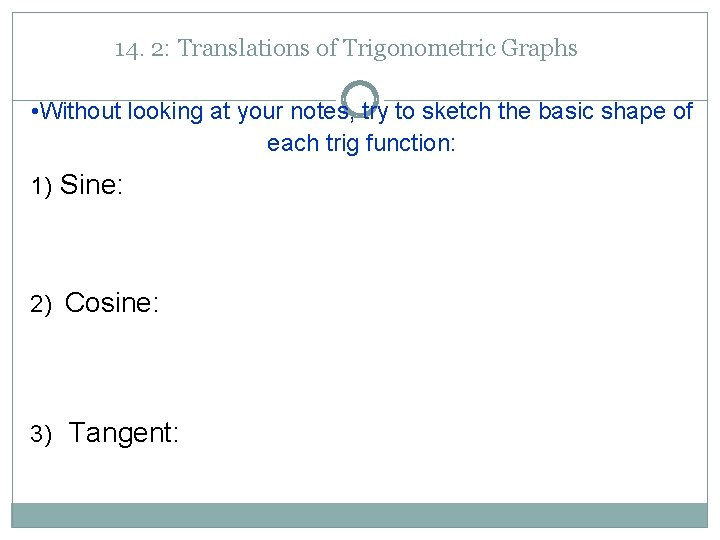14. 2: Translations of Trigonometric Graphs • Without looking at your notes, try to sketch the basic shape of each trig function: 1) Sine: 2) Cosine: 3) Tangent:More Transformations ØWe have seen two types of transformations on trig graphs: vertical stretches and horizontal stretches. ØThere are three more: vertical translations (slides), horizontal translations, and reflections (flips).More Transformations ØHere is the full general form for the sine function: ØJust as with parabolas and other functions, h and k are translations: Ø h slides the graph horizontally (opposite of sign) Ø k slides the graph vertically ØAlso, if a is negative, the graph is flipped vertically.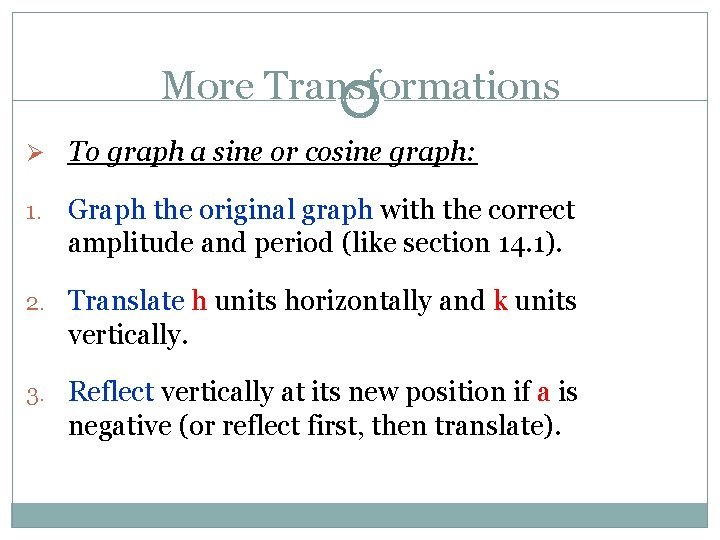More Transformations Ø To graph a sine or cosine graph: 1. Graph the original graph with the correct amplitude and period (like section 14. 1). 2. Translate h units horizontally and k units vertically. 3. Reflect vertically at its new position if a is negative (or reflect first, then translate).Examples Ø Describe how each graph would be transformed: 1. 2. 3.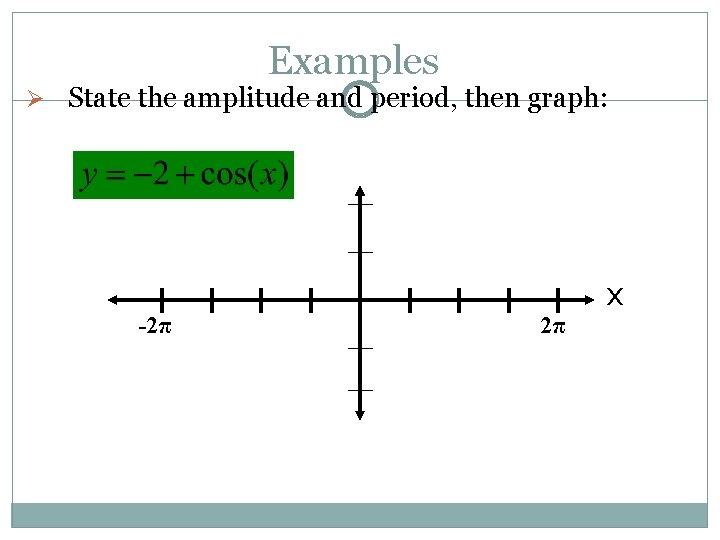Examples Ø State the amplitude and period, then graph: -2π 2π xExamples Ø State the amplitude and period, then graph: -2π 2π xØ Examples State the amplitude and period, then graph: -2π 2π x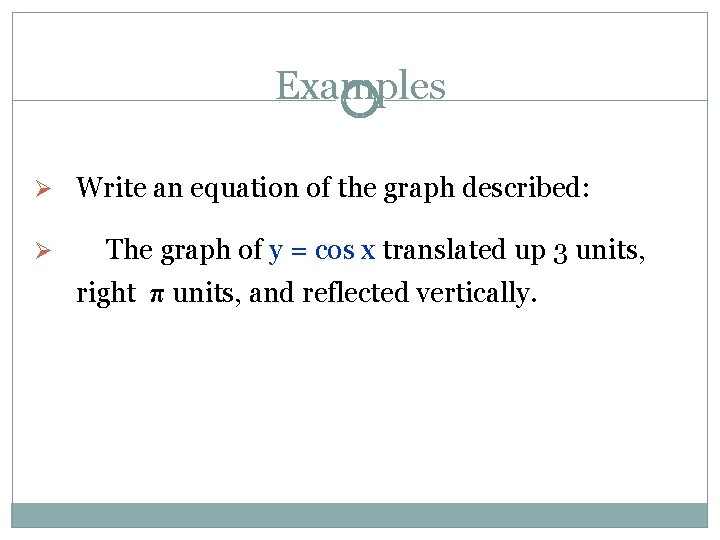Examples Ø Write an equation of the graph described: Ø The graph of y = cos x translated up 3 units, right π units, and reflected vertically.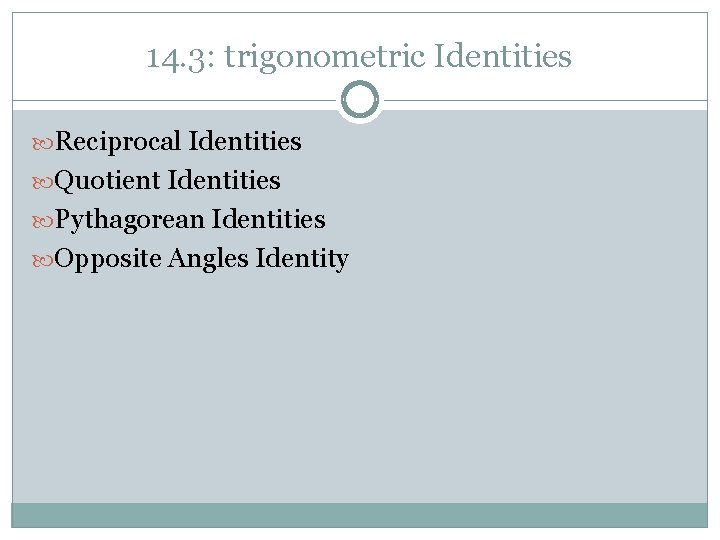14. 3: trigonometric Identities Reciprocal Identities Quotient Identities Pythagorean Identities Opposite Angles IdentitySome Vocab Identity: a statement of equality between two expressions that is true for all values of the variable(s) 2. Trigonometric Identity: an identity involving trig expressions 3. Counterexample: an example that shows an equation is false. 1.Prove that sin(x)tan(x) = cos(x) is not a trig identity by producing a counterexample. You can do this by picking almost any angle measure. Use ones that you know exact values for: 0, π/6, π/4, π/3, π/2, and π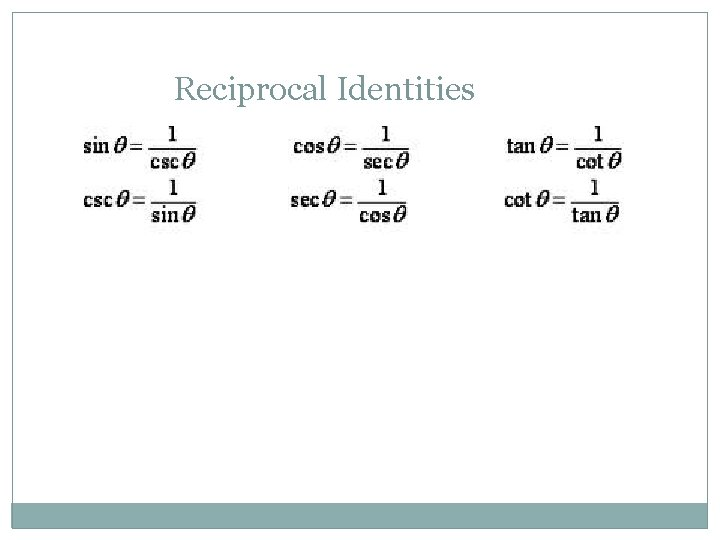Reciprocal IdentitiesQuotient Identities Why?Do you remember the Unit Circle? What is the equation for the unit circle? x 2 + y 2 = 1 • What does x = ? What does y = ? (in terms of trig functions) sin 2θ + cos 2θ = 1 Pythagorean Identity!Take the Pythagorean Identity and discover a new one! Hint: Try dividing everything by cos 2θ sin 2θ + cos 2θ = 1. cos 2θ tan 2θ + 1 = sec 2θ Quotien t Identity another Pythagorean Identity Reciproc al Identity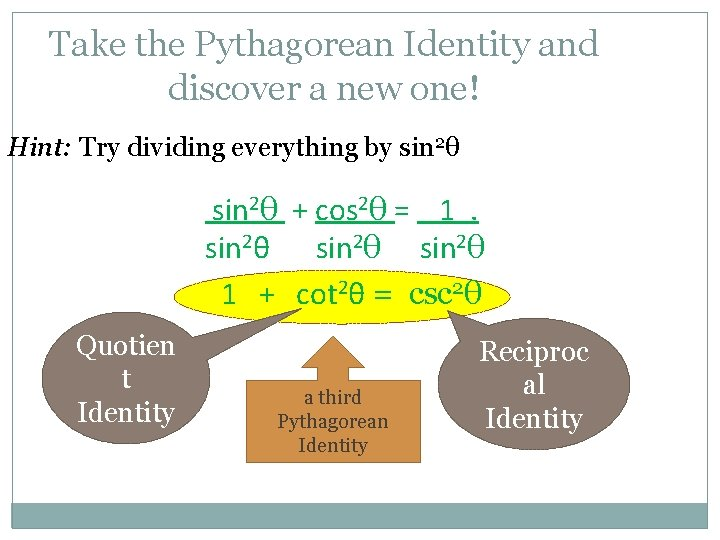Take the Pythagorean Identity and discover a new one! Hint: Try dividing everything by sin 2θ + cos 2θ = 1. sin 2θ 1 + cot 2θ = csc 2θ Quotien t Identity a third Pythagorean Identity Reciproc al IdentityOpposite Angle Identities sometimes these are called even/odd identities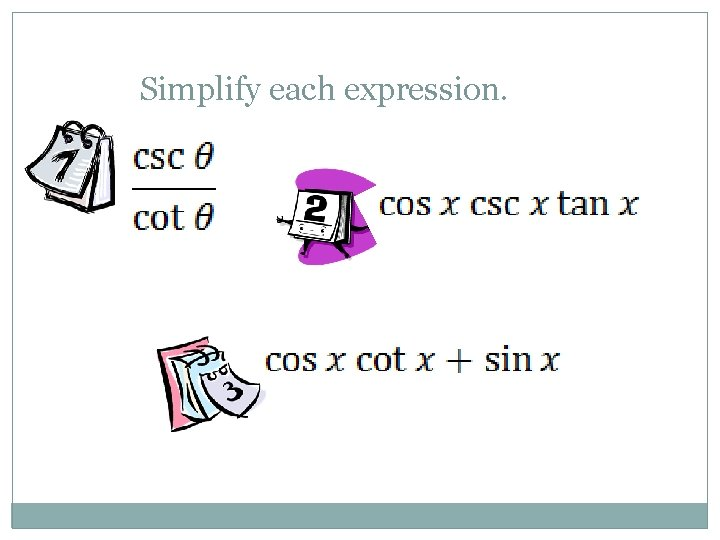Simplify each expression.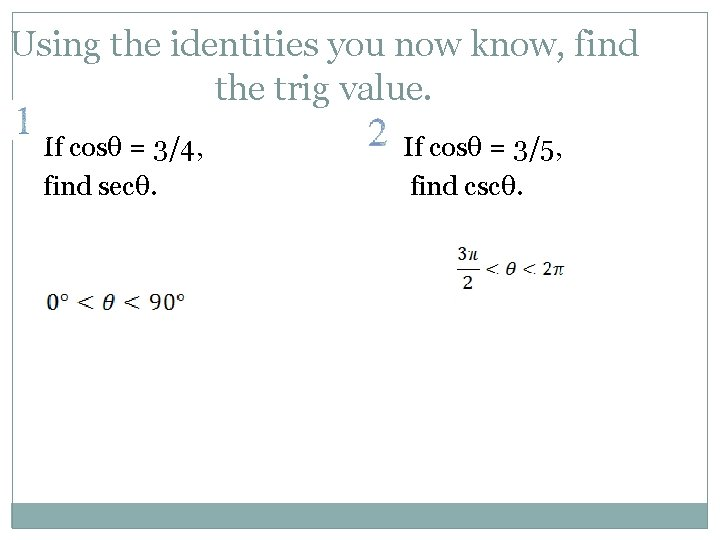Using the identities you now know, find the trig value. If cosθ = 3/4, find secθ. If cosθ = 3/5, find cscθ.sinθ = -1/3, 180 o < θ < 270 o; find tanθ secθ = -7/5, π < θ < 3π/2; find sinθ– Similarities and Differences a) How do you find the amplitude and period for sine and cosine functions? b) How do you find the amplitude, period and asymptotes for tangent? c) What process do you follow to graph any of the trigonometric functions?